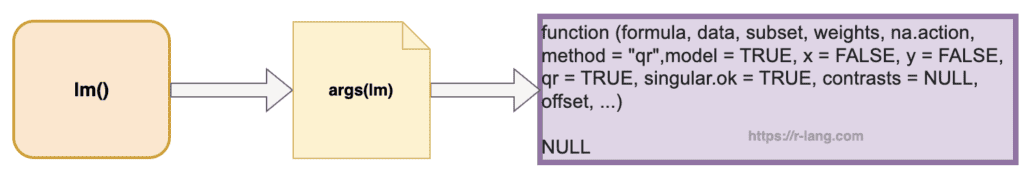# How to Use the args() Function in R

The args() function in R is used to return the required arguments or parameters of a given function. It is helpful to see what arguments a function accepts without looking up its documentation.

## Syntax

``args(name)``

## Parameters

name: It represents the name of the function.

## Return value

The args() function returns the default values.

## Visual Representation of args()## Example 1: Viewing the arguments of a base R function

``````print(args(lm))
``````

Output

``````function (formula, data, subset, weights, na.action, method = "qr",
model = TRUE, x = FALSE, y = FALSE, qr = TRUE, singular.ok = TRUE,
contrasts = NULL, offset, ...)
NULL
``````

## Example 2: Viewing the arguments of a custom function

Suppose you have defined a custom function.

``````main_function <- function(x, y, z = 10) {
return(x + y + z)
}

args(main_function)``````

Output

``````function (x, y, z = 10)
NULL``````

## Example 3: Checking if a function has a specific argument

You can use the args() function in combination with other functions to check if a specific argument exists for a given function.

For instance, let’s check if the lm() function has an argument named data:

``"data" %in% names(args(lm))``

This will return TRUE, indicating that the lm() function does have an argument named data.

That’s all!

## Applications of args() function

1. Before releasing a new version, use the args() function to check that essential function arguments remain consistent, ensuring backward compatibility.
2. You can check if a function accepts the necessary arguments before making the call, preventing potential errors in your Shiny app.
3. You can use the args() function to check the arguments of the internal function and ensure that your wrapper correctly passes the arguments.

## Related posts

How to Read Command Line Parameters in R